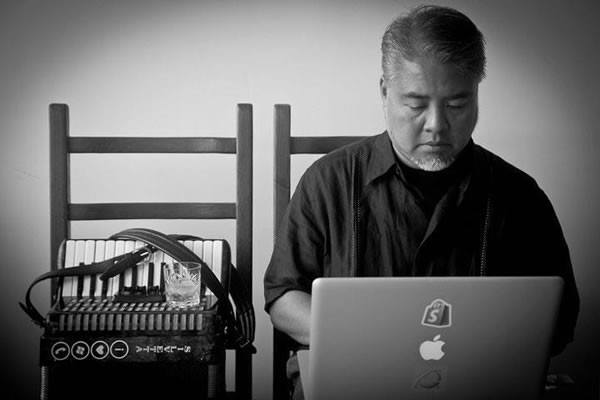Categories

# Happy new year! Now let’s do some math with the number 2017.“2017” in balloons at my local Publix. This is as well as I could arrange and photograph them before the people in charge of the balloons shoo’d me away.

Happy 2017!

Rather than write about whether or not 2016 was a terrible year (John Oliver summed it up far better than I could; note that there’s swearing) or a great one (hey, I landed a great new job featuring a significant pay raise and took Anitra to both the Philippines and the United Kingdom), I spent an hour this morning putting together a set of mini-programs that explore some of the interesting properties of the number 2017.That’s right: I spent the morning after a pleasantly boozy New Year’s Eve doing programming and math. Because that’s how I roll.

I was inspired by TJ Wei, who posted an article titled 2017 is not just another prime number, in which he states the following about the number 2017:

• 2017π (rounds to nearest integer) is a prime
• 2017e (rounds to nearest integer ) is a prime.
• The sum of all odd primes up to 2017 is a prime number, i.e. 3+5+7+11+…+2017 is a prime number.
• The sum of the cube of gap of primes up to 2017 is a prime number. That is (3-2)^3 + (5-3)^3 + (7-5)^3 + (11-7)^3 + … + (2017-2011)^3 is a prime number.
• The prime number before 2017 is 2017+(2-0-1-7), which makes it a sexy prime, and the prime after 2017 is 2017+(2+0+1+7). 2017 itself is of course equal to  2017+(2*0*1*7)
• Insert 7 into any two digits of 2017, it is still a prime number, i.e. 27017, 20717, 20177 are all primes. Plus, 20177 is also a prime number
• Since all digits of 2017 is less than 8, it can be viewed as an octal. 2017 is still a prime number as an octal.
• 2017 can be written as a sum of three cubes of primes, i,e,  p^3 +q^3 +r^3 for some primes p, q, r.
• 2017 can be written as a sum of cubes of five distinct integers.
• 2017 can be written as  x^2+y^2, x^2+2y^2, x^2+3y^2, x^2+4y^2 x^2+6y^2, x^2+7y^2, x^2+8y^2, x^2+9y^2 (for positive integers x, y)
• 20170123456789 is also a prime
• the 2017th prime number is 17539 and 201717539 is also a prime.
• Let p=2017, then both (p+1)/2 and (p+2)/3 are prime numbers.
• The first ten digits of the decimal expansion of the cubic root of 2017 contains all different digits 0~9. 2017 is the least integer has this property.
• 2017 = 2^11 – 11th prime

In case you’d forgotten your math, a prime number is a whole number that is:

• greater than 1
• has only 2 whole-number factors (that is, it can be divided by only 2 whole numbers): 1 and itself

2, 3, 5, and 7 are primes; 4, 6, 8, and 9 are not.

Prime numbers have always been interesting to mathematicians, and they have all sort of interesting uses, including cryptography, which is useful for keeping things like your files, communications, and your e-commerce transactions secure:

Rather than take Wei’s word on his fun facts about 2017, I decided to test a few of his statements using SageMath. The “Sage” in SageMath is short for “System for Algebra and Geometry Experimentation) and it’s an online system that lets you perform calculations and share your results.

For the statements about the number 2017 that I tested, Wei is correct! Perhaps I’ll test the others later and update my post.

You can see my mini-programs here; feel free to get your own SageMath account (it’s free) and test his other statements!

As for me, it’s time to head off to the gym. Happy new year, everybody!

## Also worth watching

Here’s funny math guy Matt Parker, the Standup Mathematician, sharing 17 facts about the number 2017 in 2 minutes, 17 seconds: